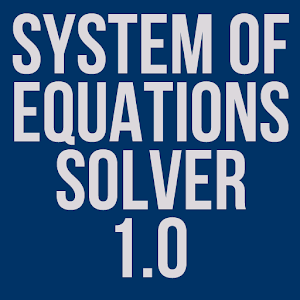# Equation Solver (System, 3&2)This application will solve a system of linear equations with either three variables or two variables. For example, let's say you have the system of equations:2x - y = -15x + 4y = 30This application will either solve for x & y, or tell you that there is no solution or an infinite number of solutions.This app will also solve system of equations with three variables, x, y, & z. Let's say you have a system of equations:x + y + z = 102x - y - 3z = 16.5x + 2y + 4z = 6This application will solve for x, y, and z or tell you when the system is unsolvable.Please leave constructive feedback and I'll address those issues as soon as I can. 应用大小：:0.060546875 MB 价格: \$ 0 浏览人数:40 排名: 3.20